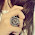### Calculation Filters

With a calculation filter, Tableau performs a calculation on the selected dimension members. By definition, a calculation filter exists when:
• The dimension is used only on the Filters shelf (that is, the dimension does not appear on other shelves).
• The filter is defined to include multiple values.
The calculation that is performed depends on the data source. For relational data sources, as shown below, the calculation matches the aggregation for each measure used in the view. For multidimensional data sources, as shown in Calculation Filters: Multidimensional Data Source, the calculation is always a summation. In Tableau, multidimensional data sources are supported only in Windows.
Consider the following view.On the Columns shelf, the Profit measure is aggregated as a summation and the Order Quantity measure (hidden beneath the tooltip) is aggregated as an average. These measures are displayed with the Ship Mode and Container dimensions (on the Rows shelf). A filter that limits the view to two members of the Order Priority dimension is applied to the data:Tableau automatically applies the appropriate calculation to the members of the filter based on the aggregation of each measure. Therefore, a summation is performed for Profit and an average is performed for Order Quantity.
For example, the tooltip shows the data for Jumbo Drums delivered by truck. The average order quantity is 25.18. This number was calculated by averaging the order quantities for all the rows that have a Critical or High order priority. Similarly, the sum of profit is \$682,196. This number was calculated by summing the profit for all the rows that have a Critical or High order priority.

1.Hello,

plz check out the list of dofollow social bookmarking sites with high PR are
http://www.hookupromance.com/
http://romanceden.com/
http://hookuploves.com/
http://hookupmilky.com/
2.